Courses

# NEET Previous year questions (2014-20): System of Particles and Rotational Motion Class 11 Notes | EduRev

## Class 11 : NEET Previous year questions (2014-20): System of Particles and Rotational Motion Class 11 Notes | EduRev

The document NEET Previous year questions (2014-20): System of Particles and Rotational Motion Class 11 Notes | EduRev is a part of the Class 11 Course Physics Class 11.
All you need of Class 11 at this link: Class 11

Q.1. Find the torque about the origin when a force of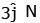acts on a particle whose position vector is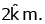A: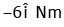B: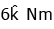C: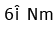D: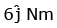Ans:
A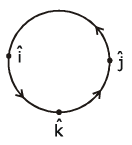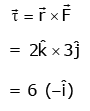Q.2. Two particles of mass 5kg and 10 kg respectively are attached to the two ends of a rigid rod of length 1m with negligible mass. The centre of mass of the system from the 5kg particle is nearly at a distance of:     
A: 67 cm
B: 80 cm
C: 33 cm
D: 50 cm
Ans:
A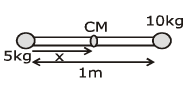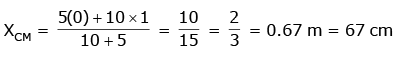Q.3. A solid cylinder of mass 2 kg and radius 4 cm is rotating about its axis at the rate of 3 rpm. The torque required to stop after 2π revolutions is    
A: 2 × 10-6 N m
B: 2 × 10-3 N m
C: 12 × 10-4 N m
D: 2 × 106 N m
Ans:
A
Solution: Work energy theorem.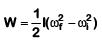θ = 2π revolution
= 2π × 2π = 4π2 rad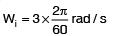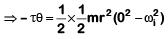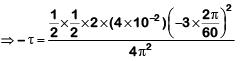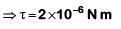Q.4. Two particles A and B are moving in uniform circular motion in concentric circles of radii rA and rB with speed vA and vB respectively. Their time period of rotation is the same. The ratio of angular speed of A to that of B will be:    
A: rA : rB
B: vA : vB
C: rB : rA
D: 1 : 1
Ans:
D
Solution: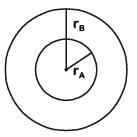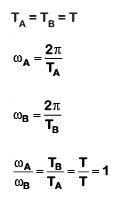Q.5. Three objects, A : (a solid sphere), B : (a thin circular disk) and C = (a circular ring), each have the same mass M and radius R. They all spin with the same angular speed ω about their own symmetry axes. The amounts of work (W) required to bring them to rest, would satisfy the relation:-    
A: WC > WB > WA
B: WA > WB > WC
C: WB > WA > WC
D: WB > WA > WC
Ans:
A
Solution: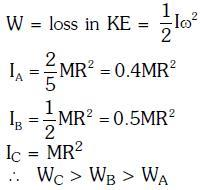Q.6. The moment of the force,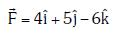at (2, 0, –3), about the point (2, –2, –2), is given by:-    
A: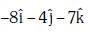B: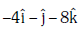C: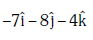D: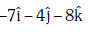Ans:
D
Solution: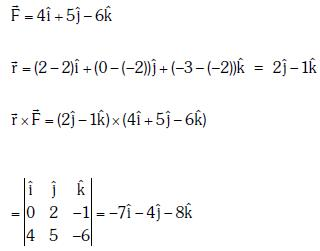Q.7. A solid sphere is in rolling motion. In rolling motion a body possesses translational kinetic energy (Kt) as well as rotational kinetic energy (Kr) simultaneously. The ratio Kt : (Kt + Kr) for the sphere is    
A: 7 : 10
B: 5 : 7
C: 10 : 7
D: 2 : 5
Ans:
B
Solution: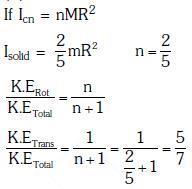Q.8. A solid sphere is rotating freely about its symmetry axis in free space. The radius of the sphere is increased keeping its mass same. Which of the following physical quantities would remain constant for the sphere ?    
A: Angular velocity
B: Moment of inertia
C: Rotational kinetic energy
D: Angular momentum
Ans:
D
Solution: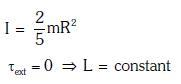Q.9. A rope is wound around a hollow cylinder of mass 3 kg and radius 40 cm. What is the angular acceleration of the cylinder if the rope is pulled with a force of 30 N ?    
C: 5 m/s2
D: 25 m/s2
Ans:
B
Solution: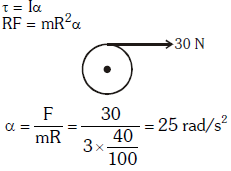Q.10. Two discs of same moment of inertia rotating about their regular axis passing through centre and perpendicular to the plane of disc with angular velocities ω1 and ω2. They are brought into contact face to face coinciding the axis of rotation. The expression for loss of energy during this process is:-    
A: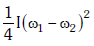B: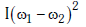C: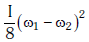D: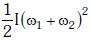Ans:
A
Solution: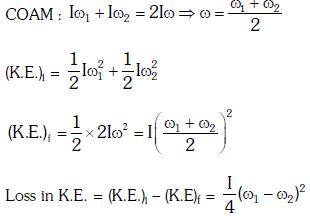Q.11. Which of the following statements are correct ?    
(a) Centre of mass of a body always coincides with the centre of gravity of the body
(b) Central of mass of a body is the point at which the total gravitational torque on the body is zero
(c) A couple on a body produces both translational and rotation motion in a body
(d) Mechanical advantage greater than one means that small effort can be used to lift a large load
A: (a) and (b)
B: (b) and (c)
C: (c) and (d)
D: (b) and (d)
Ans:
D
Solution: Centre of mass may lie on centre of gravity net torque of gravitational pull is zero about centre of mass.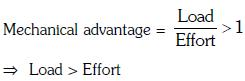Q.12. From a disc of radius R and mass M1 a circular hole of diameter R1 whose rim passes through the centre is cut. What is the moment of inertia of the remaining part of the disc about at perpendicular axis, passing through the centre ?    
A: 9MR2/32
B: 15MR2/32
C: 13MR2/32
D: 11 MR2/32
Ans
: C
Solution: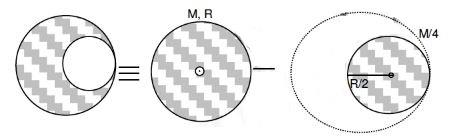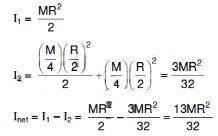Q.13. A disk and a sphere of same radius but different masses roll off on two inclined planes of the same altitude and length. Which one of the two objects gets to the bottom of the plane first ?    
A: Depends on their masses
B: Disk
C: Sphere
D: both reach at the same time
Ans:
C
Solution: Time does not depend on mass, else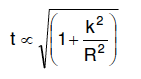K2/R2 is least for sphere and hence least time is taken by sphere

Q.14. A uniform circular disc of radius 50 cm at rest is free to turn about an axis which is perpendicular to its plane and passes through its centre. It is subjected to a torque which produces a constant angular acceleration of 2.0 rad s-2. Its net acceleration in ms-2 at the end of 2.0 s is approximately :    
A: 3.0
B: 8.0
C: 7.0
D: 6.0
Ans:
B
Solution:
The angular speed of disc increases with time, and hence centripetal acceleration also increases.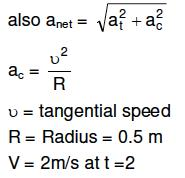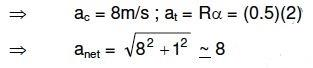Q.15. Three identical spherical shells, each of mass m and radius r are placed as shown in figure. Consider an axis XX' which is touching to two shells and passing through diameter of third shell. Moment of inertia of the system consisting of these three spherical shells about XX' axis is :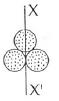A: 4 mr2
B: 11/5 mr2
C: 3 mr2
D: 16/5 mr2
Ans:
A
Solution: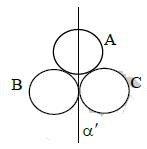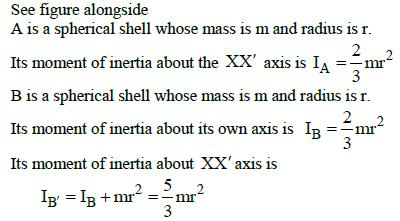Similarly the moment of inertia of the spherical shell C about the XX' axis is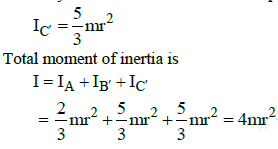Q.16. A solid cylinder of mass 50 kg and radius 0.5 m is free to rotate about the horizontal axis. A massless string is wound round the cylinder with one end attached to it and other hanging freely. Tension in the string required to produce an angular acceleration of 2 revolutions s−2 is:    
A: 78.5 N
B: 157 N
C: 25 πN
D: 50 N
Ans:
A
Solution: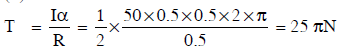Q.17. The ratio of the accelerations for a solid sphere (mass ‘m’ and radius ‘R’) rolling down an incline of angle ‘θ’ without slipping and slipping down the incline without rolling is :    
A: 2 : 5
B: 7 : 5
C: 5 : 7
D: 2 :3
Ans:
C
Solution: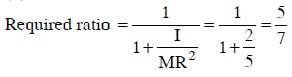Q.18. A body of mass (4m) is lying in x−y plane at rest. It suddenly explodes into three pieces. Two pieces, each of mass (m) move perpendicular to each other with equal speeds (ν). The total kinetic energy generated due to explosion is :    
A: 2 mν2
B: 4 mν2
C: mν2
D: 3/2 mν2
Ans: D
Solution
: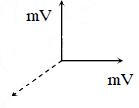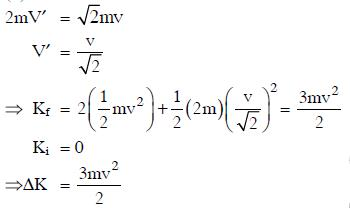Offer running on EduRev: Apply code STAYHOME200 to get INR 200 off on our premium plan EduRev Infinity!

## Physics Class 11

75 videos|312 docs|152 tests

,

,

,

,

,

,

,

,

,

,

,

,

,

,

,

,

,

,

,

,

,

;